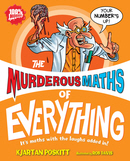#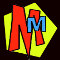How many squares are there on a chess board?

#### Oh dear - this simple question leads to some big arguments between the chess pieces! The board has 8 squares along the bottom and 8 squares up the side, so most people would say the answer is 8x8 =64. BUT suppose you count the BIG square as one, and then you also want to count up all the smaller squares - how many squares are there in total?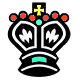If you like playing with chess boards, try The EIGHT QUEENS Puzzle!
 1 First of all the whole board makes one BIG square.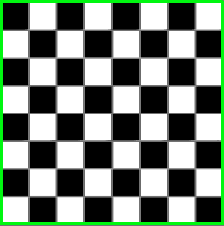+ 4 Then there are four slightly smaller squares (measuring 7x7).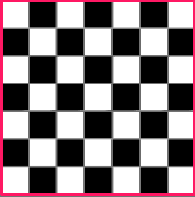We've marked where the corners go on the main board using numbers 1-4. We've outlined where the first square goes in red.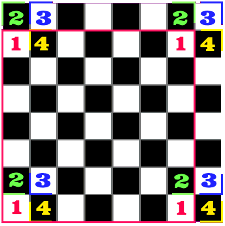+ 9 Then there are nine squares measuring 6x6.We've marked where the corners go on the main board using numbers 1-9. We've outlined where square number 2 goes in blue.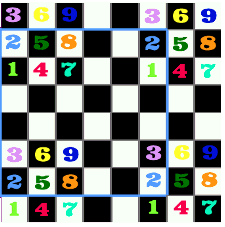When you add a list of square numbers you get the
Square Pyramid Numbers.The formula for the number of balls in a square pyramid =

### [ 2n3 + 3n2 + n ] ÷ 6

where n = the number of layers of the pyramid.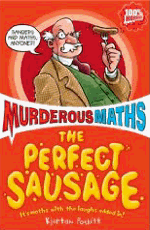The number of squares on a chessboard = the 8th pyramid number. So if you make n=8 in the formula you'll get the answer!

Find more fantastic formulas in THE PERFECT SAUSAGE. You can even find out how many rectangles are on a chess board using triangle numbers!
(The answer is T8 x T8 = 36 x 36 = 1296).

So how many squares of different sizes have we counted so far? It's 1 + 4 + 9 + ... .

You might have noticed these are the square numbers. 12=1 22=4 32=9

 Here's the next smaller size of square. How many can we fit on a chessboard? It's the next square number! 42 = + 16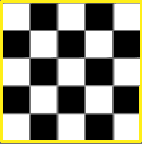Here are the other possible squares.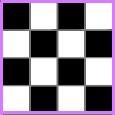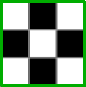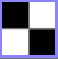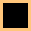Numbers of each square that will fit on the board. + 25 + 36 + 49 + 64

### So the total number of squares on the chess board = 1 + 4 + 9 + 16 + 25 + 36 + 49 + 64 = 204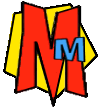The Murderous Maths of Everything